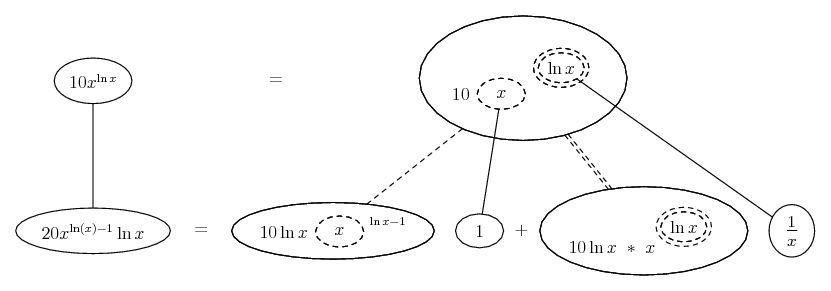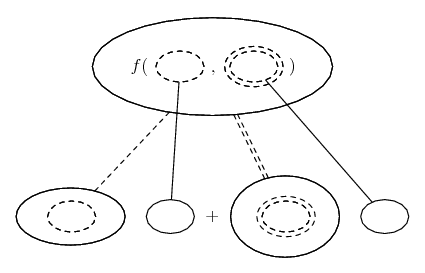1. ## Derivative

f(x)= 10x^(lnx)
How do you find the value of the derivative when x =8?

2.Originally Posted by LinaD3f(x)= 10x^(lnx)
How do you find the value of the derivative when x =8?
First you need to differentiate it. Have you done this? Then substitute x = 8 into f'(x).

3. But I'm supposed to find the value of 8 for the derivative of that function. How do I take the derivative of it?

4. You have: $\displaystyle f(x) = 10x^{ln(x)}$

Apply the chain rule:

$\displaystyle 10x^{ln (x)} \frac{d}{dx} (ln^2x)$

u=log x and n=2

$\displaystyle = 20x^{ln(x)} ln(x) \frac{d}{dx} (ln(x))$ and since the derivative of ln(x) = 1/x

you have: $\displaystyle f'(x) = 20 x^{ln(x)-1}ln(x)$

5. I don't know how to differentiate it. Can you give me a hint on how to?

6.Originally Posted by LinaD3I don't know how to differentiate it. Can you give me a hint on how to?
You have to use the chain rule. I already did it for you in my last post. Now all you need to do is to substitute $\displaystyle x=8$

7.Originally Posted by RoamYou have: $\displaystyle f(x) = 10x^{ln(x)}$

Apply the chain rule:

$\displaystyle 10x^{ln (x)} \frac{d}{dx} (ln^2x)$

u=log x and n=2

$\displaystyle = 20x^{ln(x)} ln(x) \frac{d}{dx} (ln(x))$ and since the derivative of ln(x) = 1/x

you have: $\displaystyle f'(x) = 20 x^{ln(x)-1}ln(x)$
Your answer is correct but I cannot follow the logic.

Using logarithmic differentiation:

$\displaystyle y = 10 x^{\ln x} \Rightarrow \ln y = (\ln x)^2 + \ln 10$.

Therefore $\displaystyle \frac{1}{y} \frac{dy}{dx} = \frac{2 \ln x}{x} \Rightarrow \frac{dy}{dx} = \frac{2 y \ln x}{x} = \frac{2 \left(10 x^{\ln x}\right) \ln x}{x} = 20 x^{\ln (x) - 1} \ln x$.

8. Also, just in case a picture helps convey the logic that Roam (possibly) and - Wolfram|Alpha (definitely) are using...... where... is the chain rule for two inner functions, i.e...

$\displaystyle \frac{d}{dx}\ f(u(x), v(x)) = \frac{\partial f}{\partial u} \frac{du}{dx} + \frac{\partial f}{\partial v} \frac{dv}{dx}$

As with...... the ordinary chain rule, straight continuous lines differentiate downwards (integrate up) with respect to x, and the straight dashed line similarly but with respect to the (corresponding) dashed balloon expression which is (one of) the inner function(s) of the composite expression.

_____________________________________

Don't integrate - balloontegrate!

Balloon Calculus Forum

Balloon Calculus Drawing with LaTeX and Asymptote!

#### Search Tags

derivative